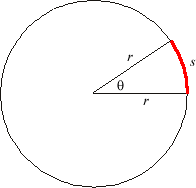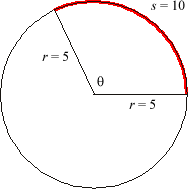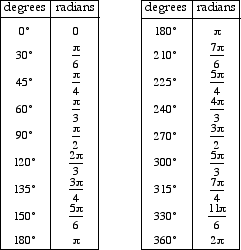## ayotzinapasomostodos.com

A unit for measuring angles. 180° = π radians, and 360° = 2π radians. The number of radians in an angle equals the number of radii it takes to measure a circular arc described by that angle.

Note: 360° equals 2π radians because a complete circular arc has length equal to 2π times the radius.Formula: θ = s/rθ = measure of the central angle in radianss = arc lengthr = radius of the circleExample:s = 10r = 5θ = 10/5 = 2 radians

Commonly used angles in degrees and radians